Next: What is convex hull? Up: Convex Polyhedron Previous: What is 0-1 polytope?   Contents

## What is the best upper bound of the numbers of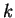-dimensional faces of a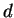-polytope with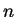vertices?

Let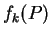denote the number of-faces of a-polytope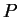, for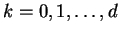.

The exact upper bound for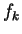in terms of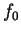and. is known, thanks to McMullen's upper bound theorem.

The convex hull of distinctpoints on the moment curve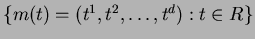in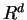is known as a cyclic polytope. It is known that its combinatorial structure (i.e. its face lattice, see Section 2.3) is uniquely determined byand. Thus we often write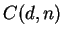to denote any such cyclic-polytope withvertices.

McMullen's Upper Bound Theorem shows that the maximum ofis attained by the cyclic polytopes.

Theorem 2 (Upper Bound Theorem)   For any-polytope withvertices,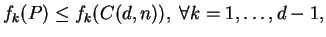holds.

The number of-faces of a cyclic polytopecan be explicitely given and thus one can evaluate the order of the upper bound in terms ofand.

Theorem 3   For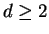and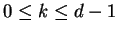,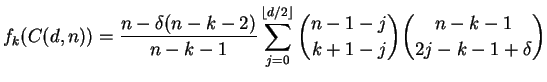where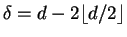. In particular,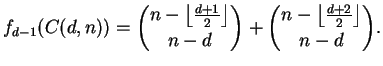(1)

For example,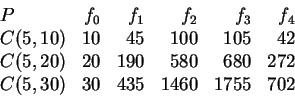The upper bound theorem can be written in dual form which gives, for example, the maximum number of vertices in a-polytope with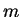facets.

Theorem 4 (Upper Bound Theorem in Dual Form)   For any-polytope withfacets,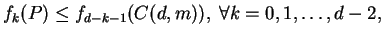holds.

The original proof of the Upper Bound Theorem is in [McM70,MS71]. There are different variations, see [Kal97,Mul94,Zie94].Next: What is convex hull? Up: Convex Polyhedron Previous: What is 0-1 polytope?   Contents
Komei Fukuda 2004-08-26